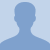# Solving Equations Containing Decimals1To solve linear equations with decimals or fractions, the first step is to get rid of the decimals or fractions. There are simple techniques to get rid of both decimals and fractions and the techniques can... To solve linear equations with decimals or fractions, the first step is to get rid of the decimals or fractions. There are simple techniques to get rid of both decimals and fractions and the techniques can be used together in case both decimals and fractions appear in the same equation. Once the equations are simplified, graph all the linear equations together.
More... Collapse
101 Views